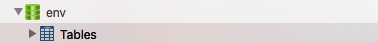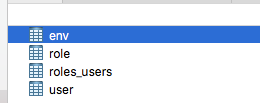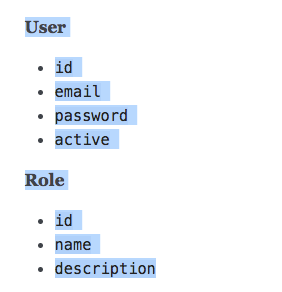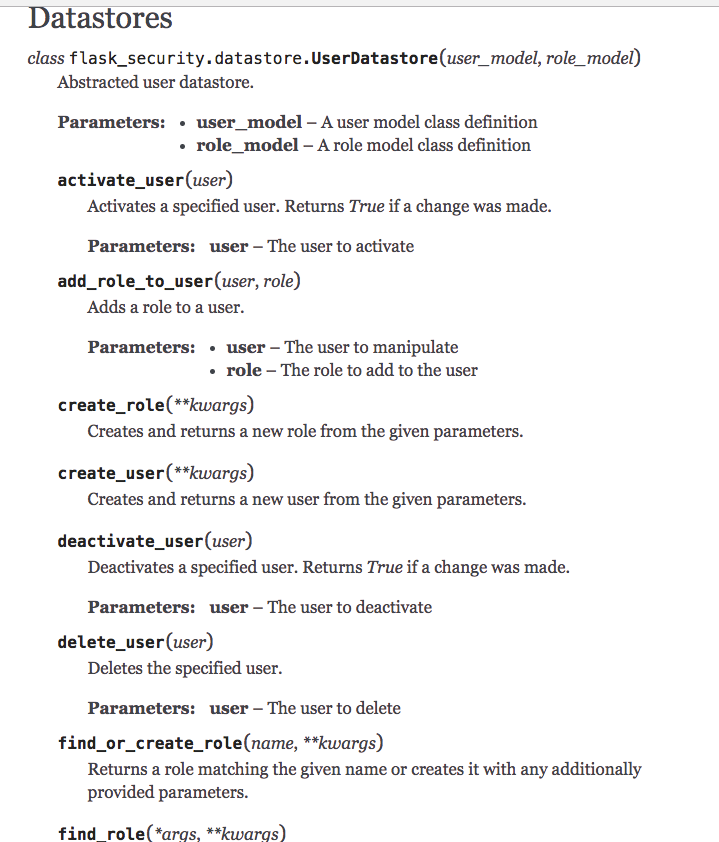## 创建数据库``````# 连接mysql数据库的配置
app.config['SQLALCHEMY_DATABASE_URI'] = 'mysql://root:root@172.27.1.221:3306/env'
``````

``````from __init__ import *

# Create database connection object
db = SQLAlchemy(app)

# Define models
roles_users = db.Table('roles_users',
db.Column('user_id', db.Integer(), db.ForeignKey('user.id')),
db.Column('role_id', db.Integer(), db.ForeignKey('role.id')))

class Role(db.Model, RoleMixin):
id = db.Column(db.Integer(), primary_key=True)
name = db.Column(db.String(80), unique=True)
description = db.Column(db.String(255))

class User(db.Model, UserMixin):

id = db.Column(db.Integer, primary_key=True)
email = db.Column(db.String(255), unique=True)
active = db.Column(db.Boolean())
confirmed_at = db.Column(db.DateTime())
roles = db.relationship('Role', secondary=roles_users,
backref=db.backref('users', lazy='dynamic'))
envs = db.relationship('Env', backref=db.backref('user'))

class Env(db.Model):

def __init__(self, name, user_id):
self.name = name
self.user_id = user_id

id = db.Column(db.Integer, primary_key=True)
name = db.Column(db.String(255), unique=True)
user_id = db.Column(db.Integer, db.ForeignKey('user.id'))
``````

``````db.create_all()
``````3 张表加一个 roles_users 的表。

## 数据库操作

``````env = Env()
env.name = 'test01'
env.user_id = '1'
db.session.commit()
``````

``````env = Env.query.filter_by(name='test01').first()
``````

``````Env.query.filter(name.endswith('01')).all()
``````

## 使用 Flask-Security 创建 User 和 Role``````from urls import db， User,  Role
user_datastore = SQLAlchemyUserDatastore(db, User, Role)
security = Security(app, user_datastore)
db.create_all()

user_datastore.create_role(name='User', description='Generic user role')
db.session.commit()
``````## 未完待续

#5 · 2017年03月10日 Author